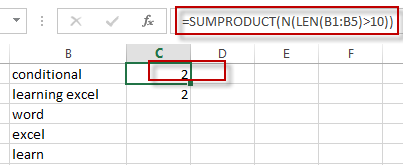# Count Number of Cells with String Length greater than X

This post will guide you how to count the total number of cells with string length in a specified range in Excel. How to get the number of cells that its length is greater than a specific number in Excel.

For Example, if you have a range of cells in B1:B5 and each cell contains one text string value have different string length. And you want to count the number of the string length of each cell is greater than number 10.

You can create a new formula based on the SUMPRODUCT function, the N function and the LEN function to check the string length of each cell and also get the total number that the string length is greater than number 10. So let’s write down the following formula:

`=SUMPRODUCT(N(LEN(B1:B5)>10))`

Or

`=SUMPRODUCT(--(LEN(B1:B5)>10))`

Then type this formula in the formula box of Cell C1, then press Enter key, you will see the result.### Related Functions

• Excel SUMPRODUCT function
The Excel SUMPRODUCT function multiplies corresponding components in the given one or more arrays or ranges, and returns the sum of those products.The syntax of the SUMPRODUCT function is as below:= SUMPRODUCT (array1,[array2],…)…
• Excel LEN function
The Excel LEN function returns the length of a text string (the number of characters in a text string).The syntax of the LEN function is as below:= LEN(text)…
• Excel N function
The Excel LEN function returns the length of a text string (the number of characters in a text string).The syntax of the LEN function is as below:= LEN(text)…